June 9, 2023

Atmospheric temperature varies with height. This well known and well understood phenomenon is called lapse rate. It describes the altitudinal temperature gradient, essentially stating that temperature decreases by 4-9.8 degrees C per km elevation. The reason for discussing this here is, that the vertical temperature gradient is often incorrectly attributed to a radiative “greenhouse effect”, where in fact it is the natural thermodynamic consequence of a gas volume in a gravitational field. As it is an adiabatic effect, there is no change of the total energy content involved.
There are many ways to explain this phenomenon. Here I want to do it in the most elementary way.

The first important assumption is the local thermodynamic equilibrium. It means that in a certain volume of air there are no macroscopic state changes of e.g. temperature or pressure. Let us assume a volume of air that is large enough so that temperature can be defined, and that is small enough so that the temperature is constant in that volume, typically called “parcel of gas”.
This volume with mass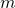is in the planetary gravitational field with gravitation constant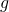. At height h it has the potential energy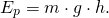and the thermal energy of this volume with heat capacity (at constant pressure)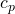and temperature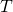w.r.t. reference temperatureis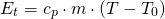The second assumption is that the system is adiabatic, i.e. there is no energy flowing into or out of the system. This means, that the sumof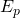and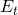is constant: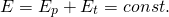Therefore the total derivative of E must be 0: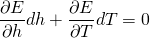From this the temperature gradient, i.e. lapse rate, follows directly: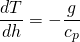What does this mean? Starting from the assumption of local conservation of energy, a molecule moving upwards loses motion energy in exchange for potential energy, must therefore get cooler by the corresponding energy amount, in other words raising a mass in the gravitational field must be paid from the motion energy, and a falling mass is accelerated, raising the temperature. Equilibrium is reached when the entropy of the system is maximal.
With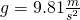andthe adiabatic lapse rate for dry air is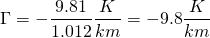If the air is humid, depending on pressure and temperature, water vapor condenses to liquid water, releasing the latent heat of 2260 J/g. This additional condensation energy reduces the lapse rate, because the “price” for potential energy can (partially) be paid from the condensation energy without decrease of temperature. The resulting moist adiabatic lapse rate is in the range -4…-9.8 K/km, depending on the humidity of the air. The global average lapse rate is -6.4 K/km.
For diving deeper into this and other details, here is an exhaustive discussion of atmospheric physics.

What has lapse rate to do with climate or greenhouse effect? In fact lapse rate explains much if not all global temperature difference between the surface of the earth and the top of troposphere withouth explicit assumptions about “forcing” or greenhouse gases (greenhouse gases are, however, relevant for the interaction with infrared radiation). The state described by lapse rate is an equilibrium state of the atmosphere without flow of energy: